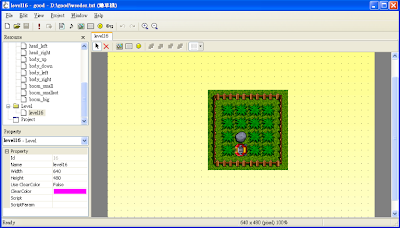## 2009年4月10日 星期五

### 實作鋤草機GamePlay

good的GamePlay使用Lua實作，所以繼續閱讀以下內容之前，我會先假設你已俱備使用Lua的基本能力，當然這也表示你對什麼是程式設計有基本的概念。good的物件類別是以Lua的Table實作的。下面的範例使用Lua的語法建立一個空的類別，也就是一個空的Table。雖然沒什麼功能，不過這樣子就能使用了，可以把它填入Script欄位。
`Level = {}`

`Level.OnStep = function(param)end`

OnStep這個函式有個叫作param的參數。這個param參數傳來的就是前面提到過的物件的Instance。

`if (Input.IsKeyPressed(Input.KEYS_LEFT)) thenend`
Input.KEYS_LEFT是一個按鍵代碼，表示我們要檢查的是Left方向鍵的狀態。除了KEYS_LEFT外，還有KEYS_RIGHT、KEYS_DOWN、KEYS_UP、KEYS_RETURN等等。

`Good.AddChild(body, head)`

`local idSpr = Good.GetSpriteId(34)``local x,y = Good.GetPos(idObj)`

`Good.SetPos(idObj, newx, newy)`

`local running = falselocal body, head = 34, 35local body_up, body_down, body_left, body_right = 9, 10, 11, 12Level = {}Level.OnStep = function(param) if (nil == param.init) then   param.init = true   Good.AddChild(body, head)   Good.SetPos(head, 0,0) end if (not running) then   if (Input.IsKeyPushed(Input.KEYS_LEFT)) then     running = true   end   return end local spd = 0.5 local x,y = Good.GetPos(body) local dir = Good.GetSpriteId(body) if (body_left == dir) then   x = x - spd elseif (body_right == dir) then   x = x + spd elseif (body_up == dir) then   y = y - spd elseif (body_down == dir) then   y = y + spd end Good.SetPos(body, x, y)end``local movement = 0local head_up, head_down, head_left, head_right = 5, 6, 7, 8Level.OnStep = function(param) ... local dir = Good.GetSpriteId(body) if (Input.IsKeyPushed(Input.KEYS_LEFT)) then   if (body_right ~= dir) then     Good.SetSpriteId(head, head_left)   end elseif (Input.IsKeyPushed(Input.KEYS_RIGHT)) then   if (body_left ~= dir) then     Good.SetSpriteId(head, head_right)   end elseif (Input.IsKeyPushed(Input.KEYS_UP)) then   if (body_down ~= dir) then     Good.SetSpriteId(head, head_up)   end elseif (Input.IsKeyPushed(Input.KEYS_DOWN)) then   if (body_up ~= dir) then     Good.SetSpriteId(head, head_down)   end end movement = movement + spd local headdir = Good.GetSpriteId(head) if (32 == movement) then   movement = 0   if (head_up == headdir) then     Good.SetSpriteId(body, body_up)   elseif (head_down == headdir) then     Good.SetSpriteId(body, body_down)   elseif (head_left == headdir) then     Good.SetSpriteId(body, body_left)   elseif (head_right == headdir) then     Good.SetSpriteId(body, body_right)   end endend`
HitTest & KillObj

`local hit = Good.FindObj(x, y, grass)`

`Good.KillObj(idObj)`

`local grass = 4local gameover = falseLevel.OnStep = function(param) ... if (32 == movement) then   Good.KillObj(Good.FindObj(x, y, grass))   ... elseif (1 == movement) then   if (head_right == headdir) then     x = x + 32   elseif (head_down == headdir) then     y = y + 32   end   local hit = Good.FindObj(x, y, grass)   if (0 >= hit) then     gameover = true   end endend`

Good模組提供了一個GenObj的方法可以讓我們動態生成一個物件。
`local idNew = Good.GenObj(idParent, idRes, script)`

`local tile = Resource.GetTileByPos(idMap, x,y)`
idMap是個地圖資源的ID，而x和y是相對於地圖左上角的座標。如果座標是有效的話，這個方法會回傳那個位置的地圖Tile的值，否則回傳0表示無效。那我們要怎麼知道我們要檢查的地圖Tile的值是多少？很簡單，打開地圖編輯器，檢視狀態列最右邊的欄位在括號中的數值就是滑鼠所指地圖Tile的值。`local rock, ground, boom_small, boom_smallest, boom_big = 59, 57, 13, 14, 15Level.OnStep = function(param) ... if (32 == movement) then   ... elseif (1 == movement) then   ...   local hit = Good.FindObj(x, y, grass)   if (0 >= hit) then     local tile = Resource.GetTileByPos(3, x - 224, y - 144)     local spr = boom_small     if (tile == rock) then       spr = boom_big     elseif (ground == tile) then       spr = boom_smallest     end     Good.GenObj(body, spr)     gameover = true   end endend`